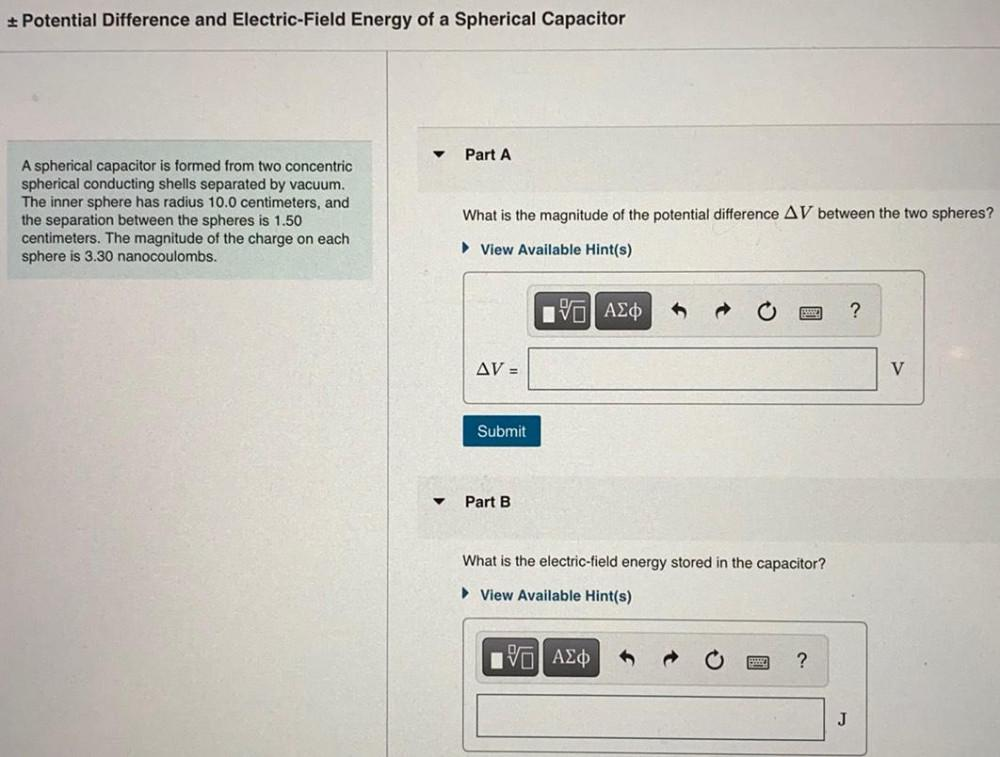Question:

# A spherical capacitor is formed from two concentric spherical conducting shells separated by vacuum. The inner sphere has radius 10.0 centimeters, and the separation between the spheres is 1.50 centimA spherical capacitor is formed from two concentric spherical conducting shells separated by vacuum. The inner sphere has radius 10.0 centimeters, and the separation between the spheres is 1.50 centimeters. The magnitude of the charge on each sphere is 3.30 nanocoulombs. a)What is the magnitude of the potential difference ΔV between the two spheres? b)What is the electric-field energy stored in the capacitor?